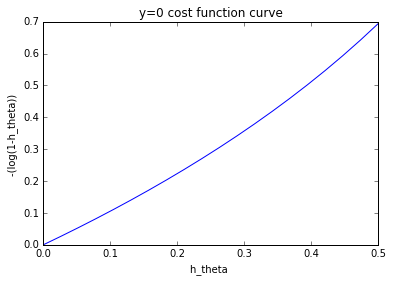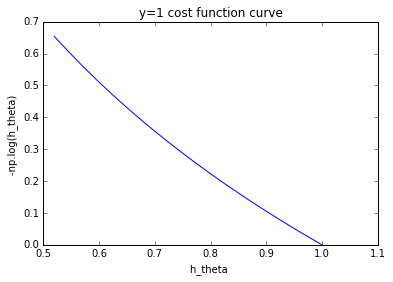# 梯度下降算法基本公式

## 常见的符号说明和损失函数

X $X$ :所有样本的特征向量组成的矩阵
x(i)$x^{(i)}$ 是第i个样本的包含的所有特征组成的向量 x(i)=x(i)1,x(i)2...,x(i)n $x^{(i)}=（x^{(i)}_1,x^{(i)}_2...,x^{(i)}_n）$
y(i)) $y^{(i)})$第i个样本的label，每个样本只有一个label， y(i) $y^{(i)}$是标量（一个数值）
hθ(x(i)) $h_\theta (x^{(i)})$ :拟合函数，机器学习中可以用多种类型的拟合函数
θ $\theta$ 是函数变量，是多个变量的向量 θ=[θ1,θ2,...] $\theta=[{\theta_1},{\theta_2},...]$
|hθ(xi)y(i)| $|{h_\theta (x^{i})-y^{(i)}}|$ :拟合绝对误差

argminθ 1mi=1m|hθ(x(i))y(i)|

argminθ 12mi=1m(hθ(xi)y(i))2

argmin $\mathop{\arg\min}$表示使目标函数取最小值时的变量值(即 θ $\theta$）值。

J(θ)= 12mi=1m(hθ(x(i))y(i))2

J(θ)=  1mi=1m|hθ(x(i))y(i)|

J(θ) $J(\theta)$不只是上面两种形式，不同的机器学习算法可以定义各种其它形式。

θj:=θjαθjJ(θ)

# 线性回归

hθ(x)=θ0+θ1x1+θ2x2+...+θnxn=θTx

J(θ)= 12mi=1m(hθ(x(i))y(i))2θT=[θ1,θ2,...]

θjJ(θ) $\frac{\partial}{\partial\theta_j}J(\theta)$求解过程还是很简单的（复合函数链式法则）。 (hθ(x(i))y(i))2 ${(h_\theta (x^{(i)})-y^{(i)}})^2$ θj ${\theta}_j$求导得到 2(hθ(x(i))y(i)) ${2(h_\theta (x^{(i)})-y^{(i)}})$ hθ(x)=θ0+θ1x1+θ2x2+...+θnxn=θTx $h_{\theta}(x)={\theta}_0+{\theta}_1x_1+{\theta}_2x_2+...+{\theta}_nx_n={\theta}^Tx$ θj ${\theta}_j$求导得到 xj ${x}_j$。因此迭代更新公式为：

θj:=θjα1mi=1m(hθ(x(i)y(i)))xj(i),xj(i)ijm

# Logistic回归

hθ(x)=11+eθTx

J(θ)=1mi=1m[(y(i)loghθ(x(i))+(1y(i))log(1hθ(x(i)))]

J(θ)=1mi=1mlog(1hθ(x(i)))

J(θ)=1mi=1mloghθ(x(i))

y=0， hθ(x) $h_{\theta}(x)$范围为[0，0.5），越接近0.5，代价越高：y=1时， hθ(x) $h_{\theta}(x)$范围为（0.5，1]，越接近0.5，代价越高：θj:=θjα1mi=1m(hθ(x(i)y(i)))xj(i),xj(i)ijm

θ:=θjα1mi=1m([hθ(x(i)y(i)))x(i)],θx(i)

# 随机梯度下降（SGD）

θj:=θjα(hθ(x(i)y(i)))xj(i)

# 参考文献

（1）Stanford机器学习—第三讲. 逻辑回归和过拟合问题的解决 logistic Regression & Regularization
http://blog.csdn.net/abcjennifer/article/details/7716281?locationNum=2
（2）机器学习入门：线性回归及梯度下降
http://blog.csdn.net/xiazdong/article/details/7950084
（3）梯度下降深入浅出
http://binhua.info/machinelearning/%E6%A2%AF%E5%BA%A6%E4%B8%8B%E9%99%8D%E6%B7%B1%E5%85%A5%E6%B5%85%E5%87%BA

11-23
12-256304-19137
09-025万+
04-012万+
05-161万+
06-271538
08-2463
04-091474
06-112005